## Wednesday, February 5, 2014

### Trigger a Matlab function with another Matlab function

Thank you for your question, Mahmoud Hasanloo. I found a solution to your problem I hope helps you. First of all, the problem is that there is a subsystem which generates a condition, that condition is read by a matlab function. The occurrence of that condition is detected by another Matlab function, and it is needed to related both Matlab functions.

The first thing that comes to my mind is that you can join the two Matlab function codes into one, so the occurrence of the condition is detected and the the condition could be executed.

Another solution could be to add a global variable as a flag to initialized the second Matlab function.

The last solution and the one I will explain deeply is to use the output of one Matlab function as the input of the second one. I propose you the next example solution:

I created a subsystem that generates a Random Integer between 0-4.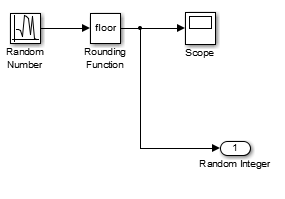Random Integer SubsystemRandom Number SourceRandom Integer Scope

Now, the occurrence of the condition that is read by a Matlab function is that the random integer generated is greater than 1 or not. In the case the occurrence is true, the condition in the second Matlab function will be executed.

function y = fcn(u)
% If input is >1, output will be true (1), otherwise output will be false (0)

if u > 1
y = 1;
else
y = 0;
endOutput =1 if input is greater than one, Output = 0 if not
Finally, the condition that is executed in the second Matlab function is that if the occurrence is true, the output will be the sumation of all past integers generated, and if the occurrence is false, the output will be reset. In order to do this sumatory, global variables are used.

Note: The integer could be saved in a global variable or could be inserted in Matlab function directly as an input variable. Sum is needed to be a global variable.

function y = fcn(u)
% If input is 1, output will the sum of the array, otherwise output will be
% 0.

global I;
global sum;

sum = sum + I;
if u
y = sum;
else
sum = 0;
y = 0;
endSumatory of the integers until output is reset
The overall system is the next one:Total system
I hope this helps to solve your problem. If there is something I undertood in a wrong way or you have any further questions do not hesitate in asking me, please. Thank you for your collaboration.## Tuesday, December 10, 2013

### Create a Library

Sometimes it is useful to have some components in a personal library. Specially for the mask or customized components. If we create a library with this type of components, they cannot be modify by an external user.

Creating a custom library is really easy. First of all, we open a new library in the Simulink enviroment and save it: File>>New library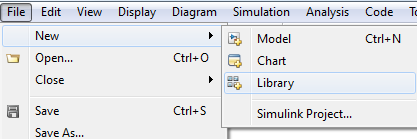New library

Then we drag the components we are interested in from the Simulink Library Browser. If we need to add the custom components we can drag them from a Simulink Model. Then we can have our customized library with our priorities and without enabling the change of the customized and mask components.

Example: Most_used_components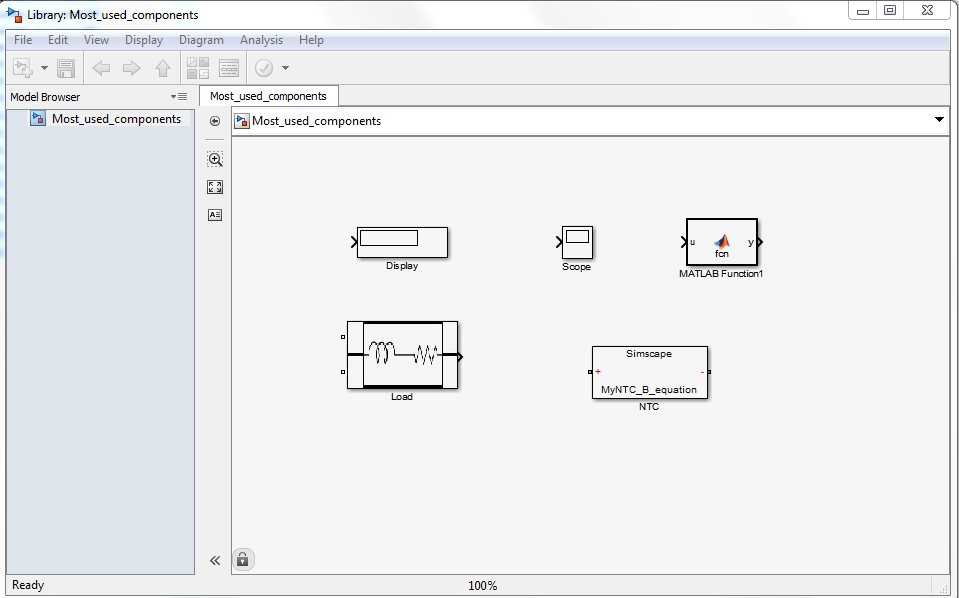Most_used_components.slx library
Notice that the extension of Simulink libraries is .slx## Wednesday, November 6, 2013

### Create a Customized Component

Hello again!

The other day I was trying to simulate a customized NTC resistor, and finally I came with the solution of creating a new custom component.

First of all, open a .ssc file in order to access to the block editor. Choose a component, for example a resistor, and open the block parameter.Blocm parameter: Resistor
Here we see the 'View source for Resistor' and if we click it we enter to the block editor:.ssc file example
Inside this block editor we can create any component.

In my case, I would like to create a NTC with B equation dependence:

Code:

component MyNTC_B_equation
Explanation that will appear in the block parameter:
%NTC  Name of the component
%Resistor with temperature dependece defined by R = R0* exp(B*(1./T-1/T0))
%Where R is the nominal resistance at the reference temperature in ohms and B is the characteristic temperature constant
% Temperature must be in Kelvin!!!

Pins that will be in our component
%component pins
nodes
p = foundation.electrical.electrical; %+:left
n = foundation.electrical.electrical; %-:right
end

variables
i = {0,'A'};
v = {0,'V'};
end

Parameters by default that the user can change:
%user interface
parameters
R0  = {33,'kOhm'}; %nominal resistance
B  = {3000, 'K'};       %characteristic temperature constant
T0 = {300,'K'} %Reference temperature
T  = {300,'K'} %Current temperature
end
%function of model
function setup
if R0 < 0
pm_error('simscape:GreaterThatOrEqualToZero','Resistance')
end
through (i, p.i, n.i);
across (v,p.v,n.v);
end

Component behaviour
%component behaviour
equations
v == R0 * exp(B*(1./T-1/T0))*i;
end
end

Note: The code is just the blue one!

Now, in order to create a library with this component, we must save the file in a folder starting with '+'. For example: '+MyComponents'.

Now, in Matlab enviroment, and in the path we have the new folder, we write:
>>ssc_build MyComponents;

Matlab will give us back a comment:
Generating Simulink library 'MyComponents_lib' in the current directory '...'

And the library will be created with the component inside it.Custom components library
Here I have two examples inside my library: NTC and R therms. The library will convert all the .ssc files to inside the folder to components.

If we select our component into a new Simulink model and we double-click it we can see the different parameters that need to be enter by an external user. We can also go to the code of the NTC.Block parameters: Custom component
Now, we can use the component in any schematic we want.

With that tool, we can create whatever we need for our examples! If you have an example I will be glad to look at it and use it in my future examples! You can use mine too!

Hope this helps!## Wednesday, October 30, 2013

### Global variables

Just the other day I was running a program in Simulink and I found a problem with global variables. The solution finally was really easy but I wanted to share it with you.

First of all, in order to initializate global variables, we can go to my previous post 'Clock Pulses Counter' where I explained how to do  it in detail.

Back to the problem, I wanted to create an array of global variables in a Matlab function block. I realized that in order to do it, I just had to initializate the Data Store Memory into an array:Array initialization Data Store Memory
Now, this global variable behaviours as an array.

Other thing I had to face was write and read a global variable out of a Matlab function block (in order to debug the code). Then I found some really interesting blocks: Data Store Read and Data Store Write, that allow us to read and write into the global variable.Data Store ReadData Store Write

With that, I wanted to implement an example with both combinations: write and read global variables in a Matlab function block and write and read the same global variables with the Data Store Read and Data Store Write blocks.

The example I thought was to generate a 3-array and the values will be incremented by 1 or by 5 depending on the block reading and writing the global variables. The result was the following: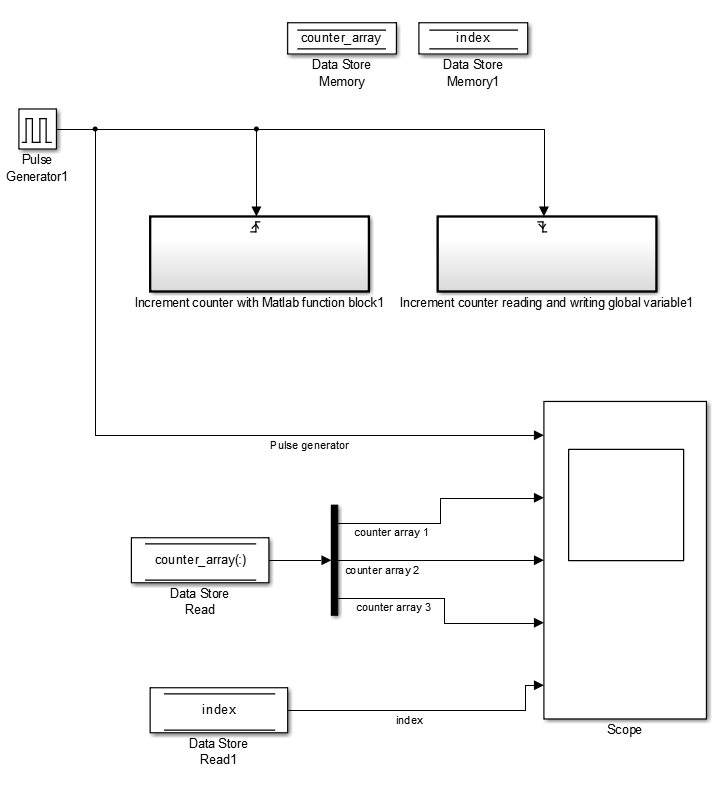Example: General Block Diagram
Then, inside the 'Increment counter with Matlab function block' we can find the following blocks:Matlab function block
By including the Trigger block or Enable block inside a subsystem we make the subsystem triggered or enabled by the outside. The code inside the block was:

function fcn

global counter_array;
global index;

if index < 3
counter_array(index) = counter_array(index) + 5; %add 5 to the value
index = index +1;
else
counter_array(index) = counter_array(index) + 5; %last index value
index = 1;
end

Inside the 'Increment counter reading and writing global variable' we can found the following blocks: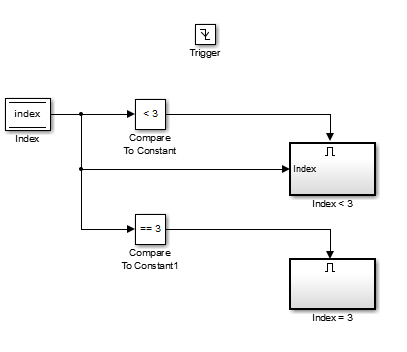Increment counter reading and writing global variable block

Now, inside the 'Index < 3' block: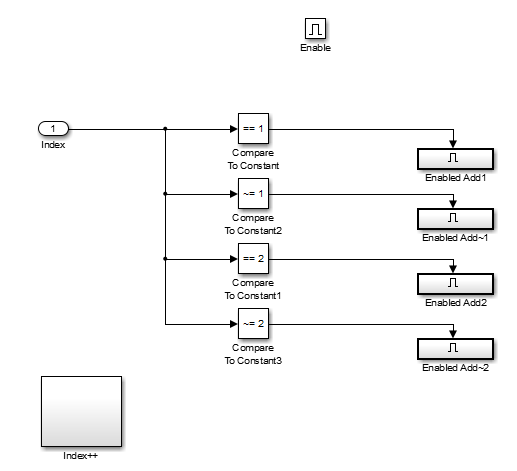Index < 3 block
Here, depending on if the index is 1 or 2 we will develop different performances.
In the case of Index ==1:Enable Add1

The value inside array(1) will be increased by 1 and also the index: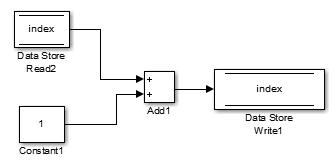Index++

In the case of index different from 1, we want to keep the array(1) and array(3) values, since we are changing index = 2, so the diagram block will be:Enable Add~1

In the case of 'Enable Add 2' and 'Enable Add~2' is the same as the blocks before but changing array(1) to array(2).
Nevertheless, in the case Index = 3, the block diagram will be the following:Index = 3
And inside index = 1 block is just as simple as write a constant = 1 inside the Data Store Variable.Index = 1

Finally, the theoretical result of that example should be:
Index = 1     Array = [0,0,0]
Index = 2     Array = [5,0,0]
Index = 3     Array = [5,1,0]
Index = 1     Array = [5,1,5]
Index = 2     Array = [6,1,5]
Index = 3     Array = [6,6,5]
Index = 1     Array = [6,6,6]
Index = 2     Array = [11,6,6]
Index = 3     Array = [11,7,6]
...

And the simulation we got was the following:Example: Final Simulation
It can be seen that it is as expected.

Notice that is much more complicated read/write global variables with the 'Data Store Read' and 'Data Store Write' blocks than with the 'Matlab function' block. However, they are very useful in order to debug our code.

Hope this is useful and any comments with problems or recommendations are welcome!! See you soon!## Wednesday, October 23, 2013

This post is related with 'Export Simulink Data to Matlab' since it is another way to compare the simulink data. In the post 'Export Simulink Data to Matlab' we created variables in Matlab in order to process the data. In this post, I will explain how to easily compare some characteristics of variables in Simulink enviroment.

Let's took the same example as in 'Export Simulink Data to Matlab':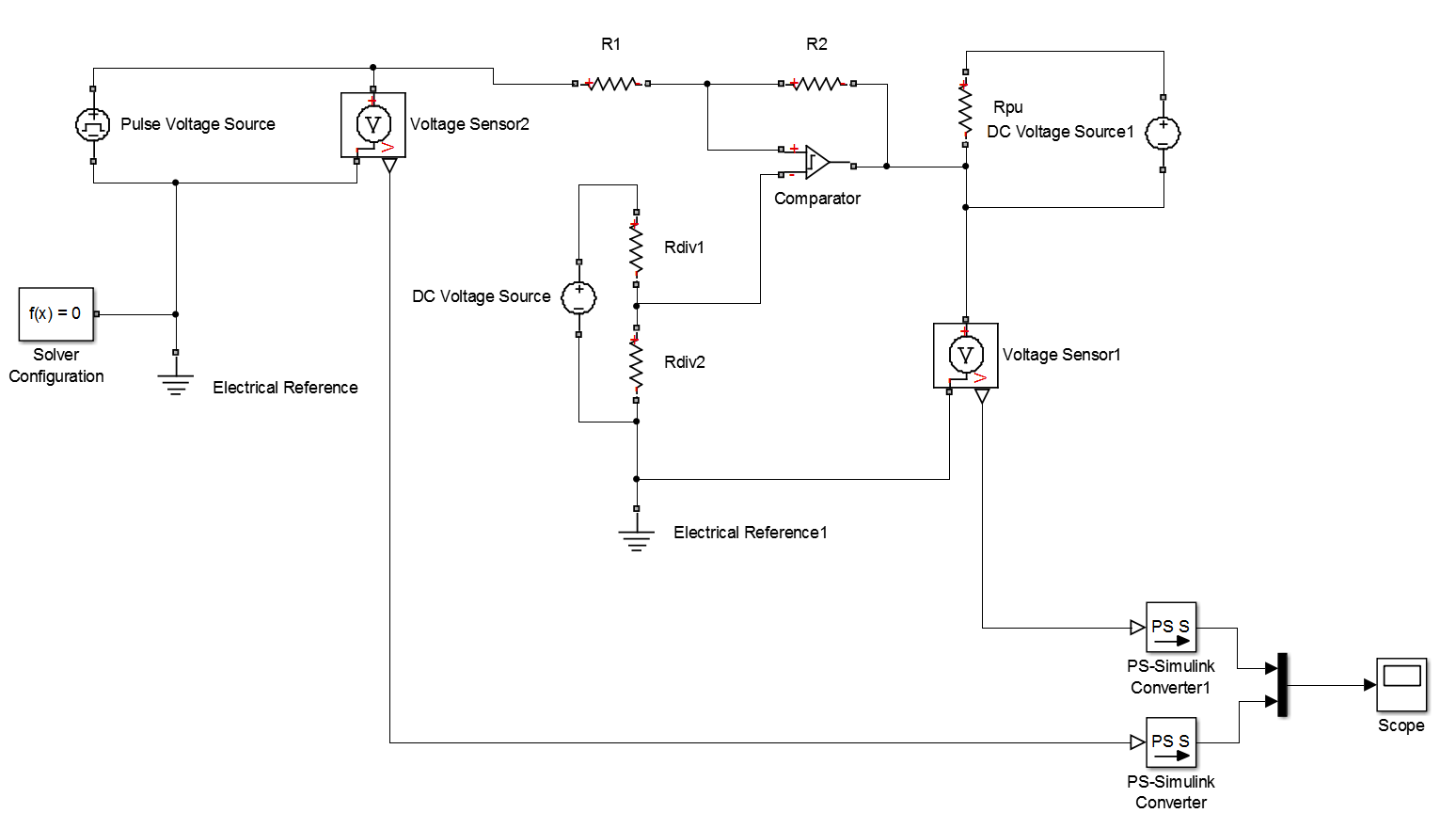Export Simulink Data to Matlab Example
Now, we want to use the 'Test Points' to collect the data and the 'Signal Logging' to export that data. (This was explained in the 'Export Simulink data to Matlab' post.Test Points and Signal Logging in signal wires.
Now, in order to initializate the Simulation Data Inspector, the buttonmust be clicked.
After running the simulation, the Simulation Data Inspector tab will appear:Simulation Data Inspector tab

And after clicking 'Simulation Data Inspector' the following window will appear:Simulation Data Inspector window
Here we can see the signals, look for a crossing points, and also compare signals with simulation with other parameters.

Example1: Let's exactly detect the crossing between the two signals we have.
In the Simulation Data Inspector, we can select the data cursor and detect the exact crossing of a run.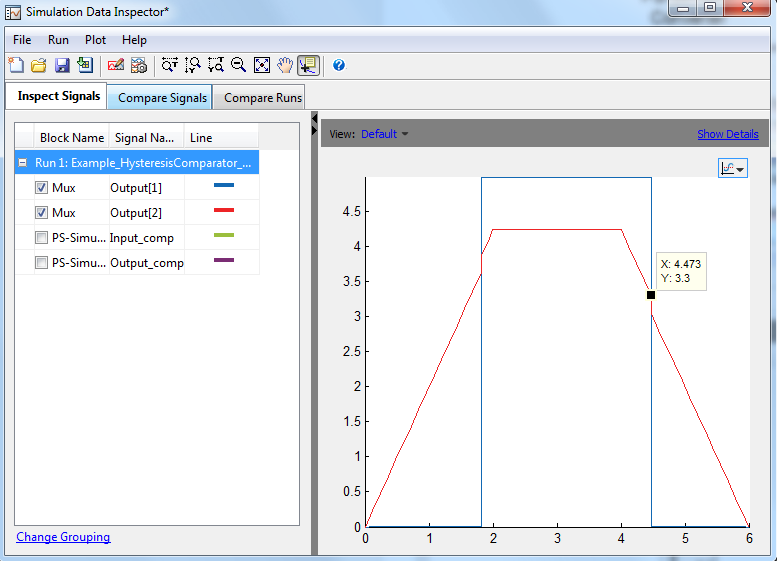Croissing point 1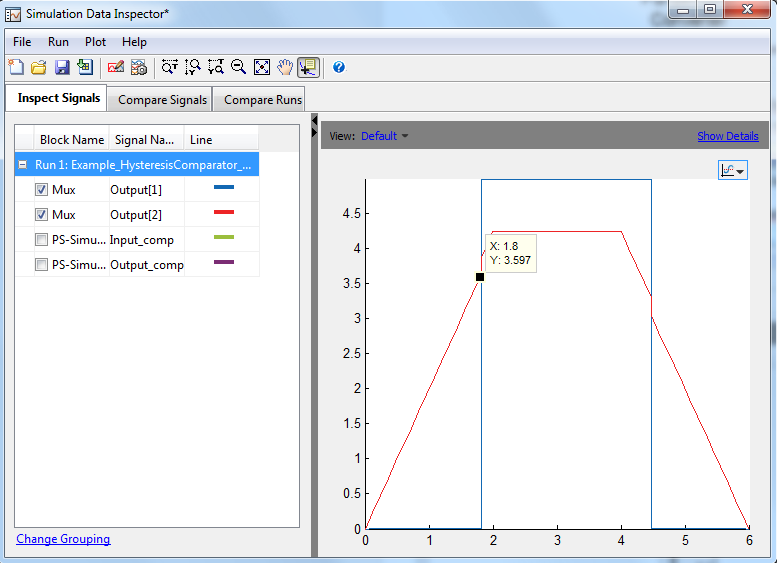Crossing point 2
Example2: Let's compare the signal by changing a resistance value:
If we change R1 from 10kOhm to 20kOhm, the output signals will appear at the window:Simulation Data Inspector - Inspect Signals
Here, we can select/deselect the signals in order to visually compare them.
Furthermore, at the compare Signals tab, we can select the signals we want to compare and the simulator will give us the difference and the tolerance between them.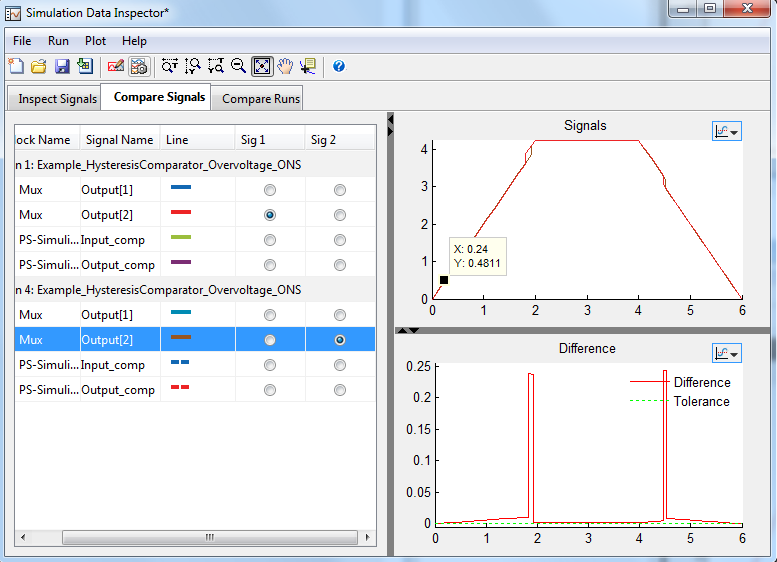Simulation Data Inspector - Compare Signals
Finally, we can select to compare runs at the Compare Runs tab and the simulator will plot the comparison between the same signals at the different runs.Simulation Data Inspector - Compare Runs
We can use this tool to process some Simulink data in an easy way. If we want to perform more difficult functions to the data, I would export it to Matlab enviroment.

Hope this help and any comment will be welcome!## Wednesday, October 16, 2013

Once you have a subsystem created, there is the possibility of mask it.

Mask a subsystem could be useful in order to customized the block and make it more accessible to an external user.

Let's take a past example to modify it and demonstrate the mask application. First of all, we take the past post 'Create a Subsystem'. If we remeber this post, I created a simple subsystem of an LR Load.Circuit inside subsystemLoad Subsystem
Now, let's mask the subsystem, customizing the previous image, and also adding some external parameters. The external parameters are useful to change the parameters on the circuit without getting inside it.

If we want to mask the subsystem, right-cliking the block, and selecting mask/create mask... or Ctrl + M when the block is selected. Then, the mask editor should open:

The mask editor allow us to change some of the subsytem options. For example, we can change the previous image of the subsystem in the first tab: Icon & Ports. Here, we can draw with Matlab plots, we can upload an image, etc.

In our case, let's take a simple image that represents our load: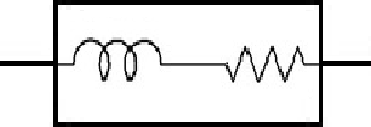RL Load
In order to upload to the subsystem, the image should be in a Matlab path or a known path. In our case, the image is inside the current directory.

Now, we program the command:

When we click apply, the changes can be seen:Subsystem with image customized
Now, we are going to add some external parameters. Let's go to the Parameters pane:Parameters tabButton to add a parameter.

There are different kind of parameter types: edit, checkbox, popup, DataTypeStr, Minimum and Maximum. In this example we are going to use edit and popup.

In the case of the popup parameter, there is a list of options that we can choose. In the case of edit, any number could be added to the subsystem.

In our case, let's limit the L values options to 330H and 390H.

Notice that in 'Type-specific options' we have specified the options we want.

Now, after clicking apply, the subsystem would appear like the following:Subsystem with mask
Now, in order to descend to the circuit, we have to click the arrow:When we normally click to the block, the window below will appear:Subsystem window

Notice that the R parameter has to be entered, and we can choose L value between 330 and 390 H.Subsystem window with popup

In order to make these parameters applicable in the circuit, we have to change the real values of R and L to our variables: 'L' and 'R':

Now, if an external user has to use the block, there is a mask to facilitate the options, like the different blocks in Simulink.

There is an initialization pane to initialize the mask block. Here we can add Matlab code that we like to run before the simulation starts.

Finally, lets add some information to the external users that can help them to understand our block. We can see a Documentation tab, where there are three sections: Mask type, Mask description, and Mask help.

Here, a explanation of the block must be added, in order to make it more understandable. The Mask type and Mask description will appear in the main window when we click the block. In we need more information, we could click the help button and the Mask help will appear:

Now, you can try with another type of parameters and options to make a more complicated subsystem. And comment the results here on the post! I will be glad to receive feedback and opinions!